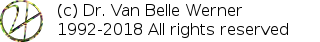Home Papers Reports Projects Code Fragments Dissertations Presentations Posters Proposals Lectures given Course notes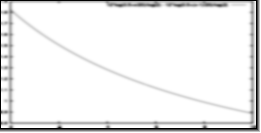# Stepwise Tempo Changes in BpmDj

Werner Van Belle1* - werner@yellowcouch.org, werner.van.belle@gmail.com

1- Yellowcouch;

Abstract :  kbpm-play provides an algorithm that automatically changes the play-rate from its current speed back to normal speed. A first version of the software relied on a linear change of pace, which turned out to be slightly wrong. In this short article we describe what went wrong and how to do it right.

Keywords:  tempo changes, psychoacoustics, preceived tempo change, bpmdj
Reference:  Werner Van Belle; Stepwise Tempo Changes in BpmDj; YellowCouch Scientific; May 2006
Files

# 1 Defining Variables

We define a number of variables necessary to investigate the problem.

• [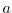] the current play speed of the song, expressed in samples/second.
• [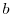] the target play-speed, expressed in samples per second.
The values placed in these two variables will lie around the sample rate.
• [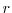] the sample rate of the original soundtrack. In BpmDj this is 44100 Hz.
We further assume a linear change of pace. E.g: every second the play-speed is updated.
• [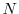] defines the number of steps necessary to reach the final speed• [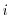] the current step. This variable ranges from 0 (at which moment the speed is supposed to be) to(at which moment the play-speed should be).
We now want to calculate the lplaying speed we need at step• [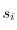] the playing speed at step.

# 2 Linear change

A first straightforward approach to determineseems linear interpolation, as follows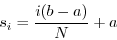The main problem we observed with this approach was that the music seemed to step up (or down) with an uneven pace. It did sound wrong. A closer investigation reveals that simple interpolation will lead to a non linear frequency change. To demonstrate this closer, we will work with a song which has only one waveform: a pure sine with frequency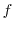.

We can now determine the frequency of that note at step(call it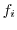) through multiplying it by the current speed () and division by its normal speed (the sample-rate).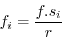(1)

Since human beings perceive frequencies in a non equally spaced manner, we need to convert this frequency to a note in a (for this purpose) equi-temporal scale. Rising one octave amounts to doubling of the note frequency. If A4 is 440 Hz, then A5 will be 880 Hz and A3 is 220 Hz. Therefore, to map the frequencyto its note number we need to take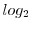(which will yield the octave number) and multiply it by 12 (to yield the note number). The perceived note at stepwill be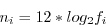(2)

Clearly, we will hear a non-linear scale. To illustrate this, let us assume that we would like to change the tempo of a song from half the playing speed (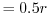) to its normal playing speed (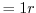) in 10 steps. Figure 1 lays out the transposition at a given time step.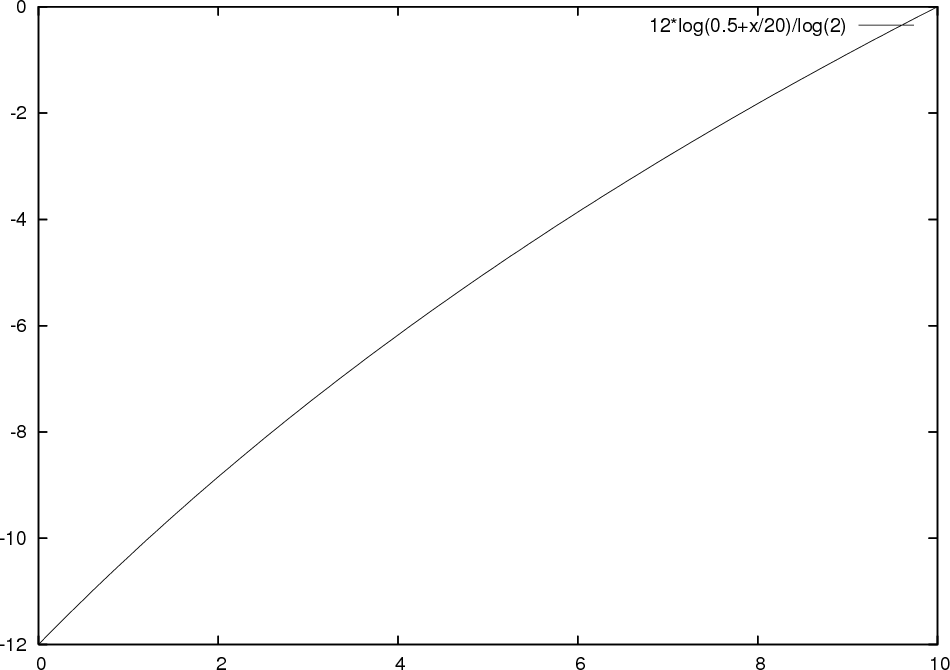The slight bend we can observe does not look as much, but when looking at the local effect by measuring the transposition at every step then the problem becomes more obvious (see figure 2)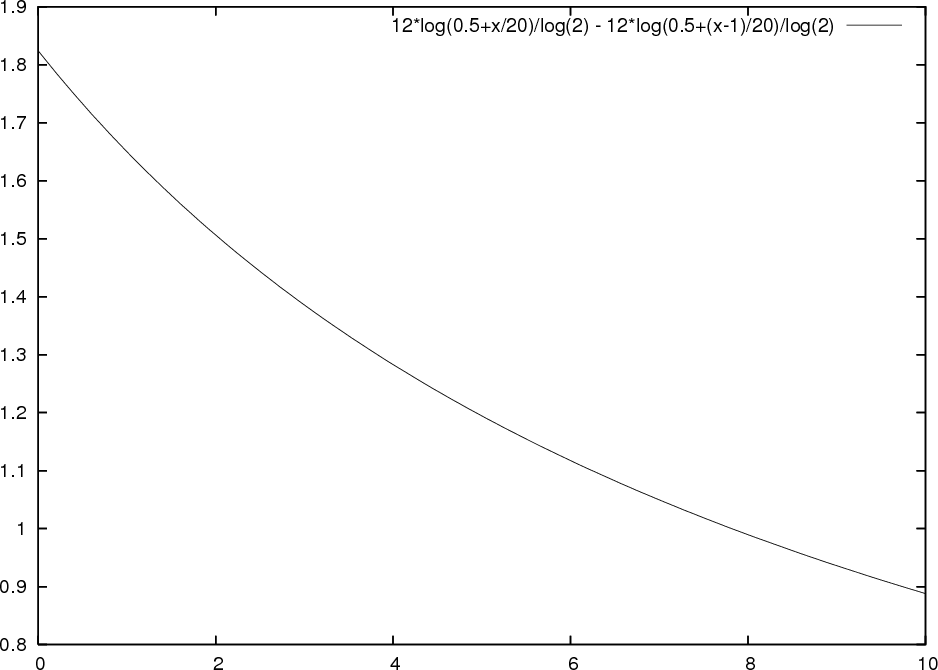# 3 Non linear change

To resolve this problem we must compensate for the logarithm and express our requirements on the note scale instead of the speed-scale. We actually want the transposition at every step to be constant (called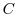). Formalized: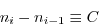According to 2 this becomes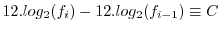. According to 1 this further gives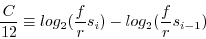Merging various constant factors (12,and) intogives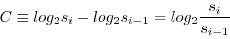and thus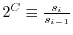, in which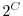will be a constant itself.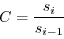In other words we need a constant multiplier for the playing speeds instead of a constant difference. By induction we get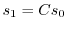,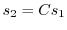,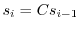which leads to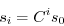(3)

We further know that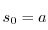and that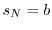, so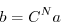Isolatinggives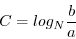(4)

Practically, filling 4 into 3 gives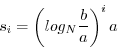To calculate the logarithm using a specific base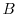(10 or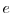for instance) use the following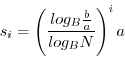http://werner.yellowcouch.org/werner@yellowcouch.org